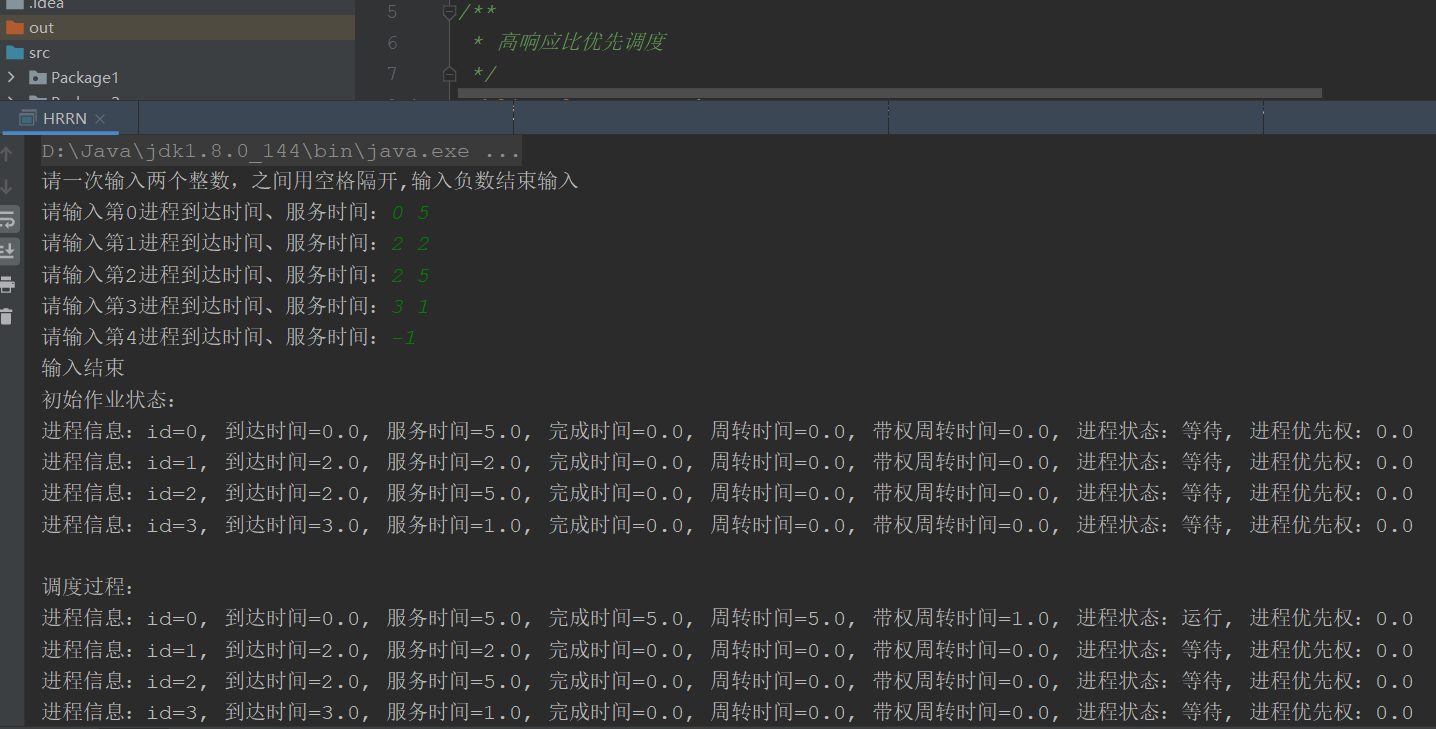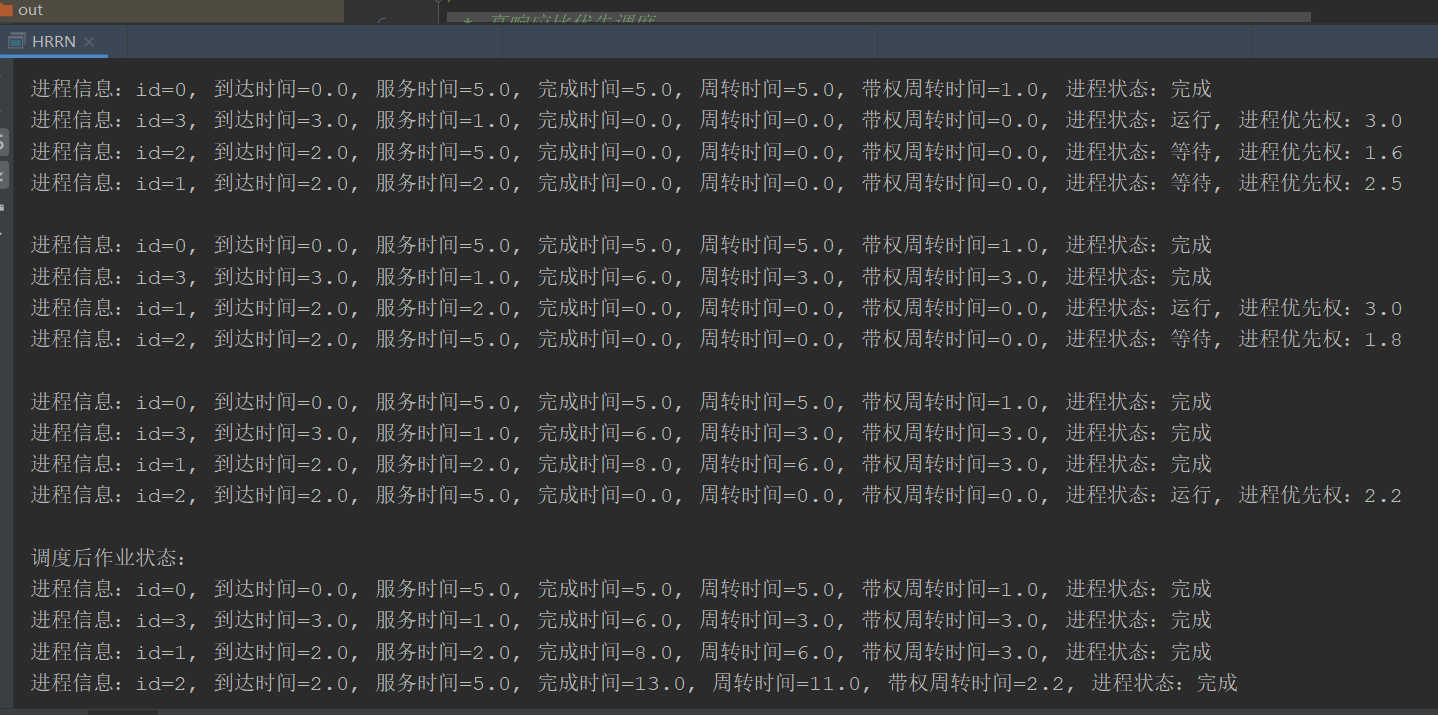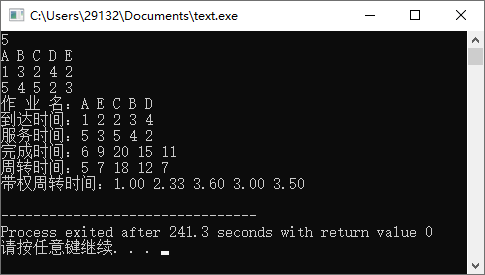• 操作系统最高响应比优先调度算法实验报告
2021-07-16 06:06:50

《操作系统最高响应比优先调度算法实验报告》由会员分享，可在线阅读，更多相关《操作系统最高响应比优先调度算法实验报告(10页珍藏版)》请在人人文库网上搜索。

1、进程调度模拟设计最高响应比优先调度算法实验报告一、实验题目与要求1、实验题目：加深对作业概念的理解。深入了解批处理系统如何组织作业、管理作业和调度作业。2、实验要求：编写程序完成批处理系统中的作业调度，要求采用响应比高者优先的作业调度算法。实现具体包括：首先确定作业控制块的内容和组成方式；然后完成作业调度；最后编写主函数，对所做工作进行测试。二、总的设计思想及语言环境、工具1、总的设计思想：最高响应比优先法(HRRN)是对FCFS方式和SJF 方式的一种综合平衡。HRRN调度策略同时考虑每个作业的等待时间长短和估计需要的执行时间长短，从中选出响应比最高的作业投入执行。 响应比R定义如下： R=。

2、(W+T)/T=1+W/T 其中T为该作业估计需要的执行时间，W为作业在后备状态队列中的等待时间。 每当要进行作业调度时，系统计算每个作业的响应比，选择其中R最大者投入执行。这样，即使是长作业，随着它等待时间的增加，W/T也就随着增加，也就有机会获得调度执行。这种算法是介于FCFS和SJF 之间的一种折中算法。由于长作业也有机会投入运行，在同一时间内处理的作业数显然要少于SJF 法，从而采用HRRN 方式时其吞吐量将小于采用SJF 法时的吞吐量。另外，由于每次调度前要计算响应比，系统开销也要相应增加。2、语言环境：计算机基本配置要求：操作系统：WIN 98/2000/XP/2003 等Wind。

3、ows平台内存：256MB及以上主存64KB(Memory)(以KB为单位分配)开发语言：Visual C+ 6.03、工具：Windows平台Visual C+ 6.0 三、数据结构与模块说明(功能与框图)作业调度的实现主要有两个问题：一个是如何将系统中的作业组织起来；另一个是如何进行作业调度。为了将系统中的作业组织起来，需要为每个进入系统的作业建立档案以记录和作业相关的信息，例如，作业名、作业所需资源、作业执行时间、作业进入系统的时间、作业信息在存储器中的位置、指向下一个作业控制块的指针等信息。这个记录作业相关信息的数据块称为作业控制块(JCB ),并将系统中等待作业调度的作业控制块组织成。

4、一个队列，这个队列称为后备队列。当进行作业调度时，从后备队列中查找选择作业。由于实验中没有实际作业，作业控制块中的信息内容只使用了实验中需要的数据。作业控制块中首先应该包括作业名；其次是作业所需资源(内存大小、打印机的数量和磁带机的数量)；采用响应比高者优先作业调度算法，为了计算响应比，还需要有作业的估计执行时间、作业在系统中的等待时间；另外，指向下一个作业控制块的指针必不可少。实验中，作业控制块及队列的数据结构定义如下：struct task string name; /*作业号*/int arrTime; /* 作业到达时间*/int serTime; /*作业要求服务时间*/int wa。

5、iTime; /*等待时间*/int begTime; /*开始运行时间*/int finTime; /*结束运行时间*/int turTime; /*周转时间*/int wTuTime; /*带权周转时间*/int priority;/*优先权*/int finish;/*是否已经完成*/JCB10;存放作业控制块的区域：#define n 10JCB jobtable10;int jobcount;将作业控制块组织成一个队列，实验中采用静态链表的方式模拟作业的后备队列，作业队列头指针定义为：int *head;实验中，内存采用可移动的动态分区管理方法，即只要内存空闲区总和比作业大就可以满足。

6、作业对内存的需求；对打印机和磁带机这两种独占设备采用静态分配法，即作业执行前必须获得所需资源，并且执行完才归还。采用响应比高者优先调度算法进行调度时，必须计算出系统中所有满足必要条件作业的响应比，从中选择响应比最高的一个作业装入主存储器分配资源。由于是实验，所以就将作业控制块出队，并输出作业名代替装入处存储器，同时修改系统的资源数量。最高响应比优先调度算法的作业调度程序流程图(如下)四、参考源程序：#include#include#include#include#include#includetypedef char string10; /* /定义string为含有10个字符元素的字符数组类。

7、型*/struct task string name; /*作业号*/int arrTime; /* 作业到达时间*/int serTime; /*作业要求服务时间*/int waiTime; /*等待时间*/int begTime; /*开始运行时间*/int finTime; /*结束运行时间*/int turTime; /*周转时间*/int wTuTime; /*带权周转时间*/int priority;/*优先权*/int finish;/*是否已经完成*/JCB10;int num; void input()int i;system(cls);printf(n请输入作业数量: );。

8、scanf(%d, &num);for(i=0;iJCBcurrent.priority )current=j;/* 找出到达时间在上一个作业完成之前，优先权高的作业*/else /* 如果作业是在上一个作业完成之后到达*/if(JCBj.arrTimeJCBcurrent.priority)current=j; /*找出服务时间比较短的一个*/return current;/*返回当前作业*/void runing(int i, int times, int pre, int staTime, int endTime)if(times=0)JCBi.begTime=JCBi.arrTime;。

9、JCBi.finTime=JCBi.begTime+JCBi.serTime; JCBi.turTime=JCBi.serTime;JCBi.wTuTime=1.0;staTime=JCBi.begTime;elseif(JCBi.arrTimeJCBpre.finTime) JCBi.begTime=JCBi.arrTime; elseJCBi.begTime=JCBpre.finTime; JCBi.finTime=JCBi.begTime+JCBi.serTime;JCBi.turTime=JCBi.finTime-JCBi.arrTime; JCBi.wTuTime=JCBi.turT。

10、ime/JCBi.serTime;if(times=num-1)endTime=JCBi.finTime;JCBi.finish=1;void print(int i,int times)if(times=0)printf( 名称 到达时间 服务时间 开始时间 完成时间 周转时间 带权周转时间n);printf(%9s%9d%9d%9d%9d%9d%9dn,JCBi.name,JCBi.arrTime,JCBi.serTime,JCBi.begTime,JCBi.finTime,JCBi.turTime,JCBi.wTuTime);void check( )int i;int staTime,。

11、 endTime, sumTurTime=0.0, sumWTuTime=0.0, aveTurTime, aveWTuTime;int current=0, times=0, pre=0; JCBpre.finTime=0; for(i=0; inum; i+)JCBi.finish=0;staTime, endTime,sumTurTime=0.0, sumWTuTime=0.0, aveTurTime, aveWTuTime;current=0; times=0; pre=0;JCBpre.finTime=0; printf(-n);for(i=0; inum; i+)JCBi.fini。

12、sh=0; staTime, endTime,sumTurTime=0.0, sumWTuTime=0.0, aveTurTime, aveWTuTime;current=0; times=0; pre=0;JCBpre.finTime=0; printf(n- HRRN -n);for(times=0; timesnum; times+)current=HRN(pre);runing(current, times, pre, staTime, endTime);print(current, times);pre=current;for(i=0; inum; i+) sumTurTime+=J。

13、CBi.turTime;sumWTuTime+=JCBi.wTuTime;aveTurTime=sumTurTime/num;aveWTuTime=sumWTuTime/num;printf(计与平均值) %9d%9d%9d%9dn,NULL,sumTurTime,aveTurTime,aveWTuTime);printf(-n);void main()char again;do system(cls); /*清屏*/ printf(please input 4 groups of datas:n);input();check();printf(Continue.(Y/N): );doagai。

14、n = getch();while(again!=Y & again!=y & again!=N & again!=n);while(again=Y | again=y);五、 运行结果与运行情况六、运行结果分析：从运行结果得到调度序列结果为：X1X2X3X1到达时间最早，服务时间也最短，其响应比最高；X2到达时间为22，但因X1早到达，所以开始时间为22，其服务时间为12，所以响应比X1小；X3到达时间最迟，其响应比最小，所以在最后。七、自我评价与总结：本次课程设计题目较为简单，主要是对优先级和最高响应比这两个算法的理解和对进程调度的功能以及进程调度算法有深入的理解。在这次的课程设计中，让我感觉较为不满意的地方是，在课程设计开始之前我对于最高响应比优先法理解不熟悉，导致了响应比的计算错误，从而加大了完成代码的时间量。对于这次出现的这个问题，使我有了对程序设计的严谨性，课本基础知识的理解程度上有了更深刻的认识，也让我明白到了基础知识的重要性。完成此次课程实际之后，我对进程调度模拟设计的各种算法有了更进一步的理解，也加深了我对于C+面向对象方面的掌握，在编写程序中所遇到的问题让我有了对操作系统有了迫切要更深层次的掌握，并操作系统这门课程实在是很重要的一门课程。

更多相关内容
• 动态优先权是指在创建进程时所赋予的优先权，是可以随进程的推进或随其等待时间的增加而改变的，以便获得更好的调度性能。引入动态优先权，并使作业的优先权随其等待时间的增长，...本实验模拟了高响应比优先调度算法
• 高响应比优先调度算法，通过C语言实现，数据结构用的是链表。操作系统的课程作业，模拟HRN，算法清楚明白，容易看懂，谢谢大家。
• 高响应比优先调度算法从文件中读取数据,操作系统实验
• java平台运行高响应比优先调度算法，如果响应相同则短作业优先。输出进程工作顺序、调度时间、周转时间、带权周转时间、平均周转时间和平均带权周转时间。压缩带有测试案例
• 比较简单，全程再小黑框里，输出有点问题，可以自己改改2这是积分版，欢迎支持，免费版去我主页下载c语言 操作系统
• 操作系统：高响应比优先调度算法（HRRN）Java代码实现

### 一、算法思想：

高响应比优先调度算法（HRRN）是一种对CPU响应比的分配的一种算法。HRRN是介于FCFS（先来先服务算法）与SJF（短作业优先算法）之间的折中算法，既考虑作业等待时间又考虑作业运行时间，既照顾短作业又不使长作业等待时间过长，改进了调度性能。

### 二、实现代码：

package Package8;

import java.util.Scanner;

/**
* 高响应比优先调度
*/
public class HRRN {

public static void main(String[] args) {

PCB[] a = new PCB;

// input方法用于输入进程信息，返回输入进程的总数
int k = input(a);

// 输出初始作业状态
System.out.println("初始作业状态：");
// 遍历输出数组
output(a, k);

// 找出最早到达的进程,优先调度
PCB min = new PCB();
min = a;
int m = 0; // 用于记录最早到达进程的id（下标）
for (int i = 0; i < k; i++) {

if (a[i].getArriveTime() < min.getArriveTime()){
min = a[i];
m = i;
} else if(a[i].getArriveTime() == min.getArriveTime() && a[i].getServiceTime() < min.getServiceTime()){
min = a[i];
m = i;
}
}
PCB temp;
temp = a;
a = min;
a[m] = temp;

System.out.println("调度过程：");
// currentTime 用于记录当前时间（最后一个已经调度的作业的完成时间）
double currentTime = 0;
// 当该进程最早到达时，直接调度
a.setCondition(1); // 调度进程，将进程状态改为运行
a.setFinishTime(a.getArriveTime() + a.getServiceTime());
a.setCyclingTime(a.getFinishTime() - a.getArriveTime());
// 将结果保留两位小数
double time = a.getCyclingTime() / a.getServiceTime();
a.setFloat_Wi(Double.parseDouble(String .format("%.2f", time)));
output(a,k); // 遍历数组
a.setCondition(2); // 进程调度完毕，将进程状态改为完成
// 记录当前时间
currentTime = a.getFinishTime();

for (int i = 1; i < k; i++) {
for (int j = i; j < k; j++) {

if (currentTime - a[j].getArriveTime() < 0){
a[j].setWaitTime(0);
} else {
a[j].setWaitTime(currentTime - a[j].getArriveTime());
}
a[j].setDibs((a[j].getWaitTime()/a[j].getServiceTime()) + 1);

}

// 找出优先权最大的进程，放到第i个位置，直接调度
PCB max;
max = a; // a的优先级默认为0
int n = 0; // 用于当前优先权最大进程的id（下标）
for (int x = i; x < k; x++) {
if (a[x].getDibs() > max.getDibs()){
max = a[x];
n = x;
} else if (a[x].getDibs() == max.getDibs() && a[x].getArriveTime() < max.getArriveTime()){
max = a[x];
n = x;
}
}
temp = a[i];
a[i] = max;
a[n] = temp;

// 调度第i个进程
a[i].setCondition(1);
output(a,k);
if (a[i].getArriveTime() > a[i - 1].getFinishTime()){
a[i].setFinishTime(a[i].getArriveTime() + a[i].getServiceTime());

} else{

a[i].setFinishTime(a[i - 1].getFinishTime() + a[i].getServiceTime());
}
a[i].setCyclingTime(a[i].getFinishTime() - a[i].getArriveTime());

// 将结果保留两位小数
time = a[i].getCyclingTime() / a[i].getServiceTime();
a[i].setFloat_Wi(Double.parseDouble(String .format("%.2f", time)));
a[i].setCondition(2);
// 记录当前时间
currentTime = a[i].getFinishTime();

// 一轮循环结束，进入下一轮循环
}

// 遍历输出数组
System.out.println("调度后作业状态：");
output(a, k);

}

/**
* 该方法用于遍历输出数组
* @param a
* @param k
*/
public static void output(PCB[] a, int k){
for (int i = 0; i < k; i++) {
System.out.println(a[i]);
if (i == k - 1){
System.out.println();
}
}
}

/**
* 该方法用于输入进程信息
* @param a
* @return 输入进程的个数
*/
public static int input(PCB[] a){

Scanner scanner = new Scanner(System.in);

int k = 0; // 初始化,k记录总进程数
System.out.println("请一次输入两个整数，之间用空格隔开,输入负数结束输入");

// 重复输入进程信息
while (true){
System.out.printf("请输入第%d进程到达时间、服务时间：", k);
// 将进程id设置为k
double arriveTime = scanner.nextDouble();
if (arriveTime < 0){
System.out.println("输入结束");
break;
}
double serviceTime = scanner.nextDouble();
a[k] = new PCB(k,arriveTime, serviceTime); // 利用构造方法初始化数据
k ++;
if (serviceTime < 0){
System.out.println("输入结束");
break;
}
}
scanner.close(); // 释放scanner

return k;
}

public static class PCB implements Package8.PCB {

private int id;
private double arriveTime; // 到达时间
private double serviceTime; // 服务时间
private double finishTime; // 完成时间
private double cyclingTime; // 周转时间
private double float_Wi; // 带权周转时间
private double waitTime; // 等待时间
private double dibs; // 优先权
private int condition; // 进程状态，0表示等待，1表示运行，2表示完成

public int getCondition() {
return condition;
}

public void setCondition(int condition) {
this.condition = condition;
}

public double getWaitTime() {
return waitTime;
}

public void setWaitTime(double waitTime) {
this.waitTime = waitTime;
}

public double getDibs() {
return dibs;
}

public void setDibs(double dibs) {
this.dibs = dibs;
}

public int getId() {
return id;
}

public void setId(int id) {
this.id = id;
}

public double getArriveTime() {
return arriveTime;
}

public void setArriveTime(double arriveTime) {
this.arriveTime = arriveTime;
}

public double getServiceTime() {
return serviceTime;
}

public void setServiceTime(double serviceTime) {
this.serviceTime = serviceTime;
}

public double getFinishTime() {
return finishTime;
}

public void setFinishTime(double finishTime) {
this.finishTime = finishTime;
}

public double getCyclingTime() {
return cyclingTime;
}

public void setCyclingTime(double cyclingTime) {
this.cyclingTime = cyclingTime;
}

public double getFloat_Wi() {
return float_Wi;
}

public void setFloat_Wi(double float_Wi) {
this.float_Wi = float_Wi;
}

public PCB() {
}

public PCB(int id, double arriveTime, double serviceTime) {
this.id = id;
this.arriveTime = arriveTime;
this.serviceTime = serviceTime;
}

@Override
public String toString() {
String condition1;
if (condition == 2){
condition1 = "完成";
return "进程信息：" +
"id=" + id +
", 到达时间=" + arriveTime +
", 服务时间=" + serviceTime +
", 完成时间=" + finishTime +
", 周转时间=" + cyclingTime +
", 带权周转时间=" + float_Wi +
", 进程状态：" + condition1;
} else {
if (condition == 1){
condition1 = "运行";
} else {
condition1 = "等待";
}

return "进程信息：" +
"id=" + id +
", 到达时间=" + arriveTime +
", 服务时间=" + serviceTime +
", 完成时间=" + finishTime +
", 周转时间=" + cyclingTime +
", 带权周转时间=" + float_Wi +
", 进程状态：" + condition1 +
", 进程优先权：" + dibs;
}
}

}
}


### 三、运行结果：展开全文java
• 高响应比优先算法 算法思想 响应比算法：(服务时间+等待时间)/服务时间 先将作业按到达时间排序，并将条件最优的作业移动到第一位，之后每次调度作业都更新后面所有作业的响应，并将最高的响应往前移。 #include...

# 高响应比优先算法

## 算法思想

响应比算法：(服务时间+等待时间)/服务时间
先将作业按到达时间排序，并将条件最优的作业移动到第一位，之后每次调度作业都更新后面所有作业的响应比，并将最高的响应比往前移。

#include<iostream>
#include<iomanip>
#include<string.h>
using namespace std;
struct work{
char name;//作业名
float arrive;//到达时间
float service;//服务时间
float finish;//完成时间
float T;//周转时间
float W;//带权周转时间
float waitTime;//等待时间
float H;//相应比
};

int main(){
int n;//作业数
struct work z;
cin>>n;
for(int i=0;i<n;i++){
cin>>z[i].name;
}

for(int i=0;i<n;i++){
cin>>z[i].arrive;
}

for(int i=0;i<n;i++){
cin>>z[i].service;
}

struct work a=z;//用一个结构体存储z或条件最优的作业的值
int min=0;//最优条件的作业的位置
for(int i=1;i<n;i++){
if(a.arrive>=z[i].arrive&&a.service>z[i].service){//判断条件更小或相等的到达时间且更短的服务时间
a=z[i];
min=i;
}
}
swap(z,z[min]);//将条件最优作业换到z位置

//从z位置开始按到达时间从小到大排序
for(int i=1;i<n-1;i++){
for(int j=i+1;j<n;j++){
if(z[i].arrive>z[j].arrive)
swap(z[i],z[j]);
}
}

//计算完成时间
z.finish=z.arrive+z.service;
for(int i=1;i<n-1;i++){
if(z[i-1].finish<z[i].arrive){//判断条件当前到达时间大于上个完成时间
z[i].finish=z[i].arrive+z[i].service;//改变完成时间算法
continue;//继续下一个循环
}

//从i开始每次循环更新等待时间和相应比
for(int l=i;l<n;l++){
z[l].waitTime=z[i-1].finish-z[l].arrive;
z[l].H=(z[l].service+z[l].waitTime)/z[l].service;
}

//从i+1开始将更优作业往前移
for(int j=i+1;j<n;j++){
if(z[j].arrive<=z[i-1].finish&&z[j].H>z[i].H){//判断条件到达时间更小或相等且响应比更大的作业
swap(z[i],z[j]);
for(int k=j;k>i+1;k--){
if(z[k].arrive<z[k-1].arrive){
swap(z[k],z[k-1]);
}
else{
break;
}
}
}
}

//按不同情况计算完成时间
if(z[i].arrive>z[i-1].finish){
z[i].finish=z[i].arrive+z[i].service;
}
else{
z[i].finish=z[i-1].finish+z[i].service;
}

//最后两个作业改变计算方式
if(i==n-2){
if(z[i+1].arrive>z[i].finish){
z[i+1].finish=z[i+1].arrive+z[i+1].service;
}
else{
z[i+1].finish=z[i].finish+z[i+1].service;
}
}
}

//计算周转时间和带权周转时间
for(int i=0;i<n;i++){
z[i].T=z[i].finish-z[i].arrive;
z[i].W=z[i].T/z[i].service;
}

for(int i=1;i<n-1;i++){
for(int j=i+1;j<n;j++){
if(z[i].arrive>z[j].arrive)
swap(z[i],z[j]);
}
}

cout<<"作 业 名：";
for(int i=0;i<n;i++){
cout<<z[i].name;
if(i!=n-1)
cout<<" ";
}
cout<<endl;

cout<<"到达时间：";
for(int i=0;i<n;i++){
cout<<z[i].arrive;
if(i!=n-1)
cout<<" ";
}
cout<<endl;

cout<<"服务时间：";
for(int i=0;i<n;i++){
cout<<z[i].service;
if(i!=n-1)
cout<<" ";
}
cout<<endl;

cout<<"完成时间：";
for(int i=0;i<n;i++){
cout<<z[i].finish;
if(i!=n-1)
cout<<" ";
}
cout<<endl;

cout<<"周转时间：";
for(int i=0;i<n;i++){
cout<<z[i].T;
if(i!=n-1)
cout<<" ";
}
cout<<endl;

cout<<"带权周转时间：";
for(int i=0;i<n;i++){
cout<<fixed<<setprecision(2)<<z[i].W;
if(i!=n-1)
cout<<" ";
}
cout<<endl;

return 0;
}展开全文• ## 例题：最高响应比优先调度算法

万次阅读 多人点赞 2020-11-05 18:43:38
高响应比优先调度算法主要用于作业调度，该算法是对FCFS调度算法和SJF调度算法的一种综合平衡，同时考虑每个作业的等待时间和估计的运行时间。在每次进行作业调度时，先计算后备作业队列中每个作业的响应，从中...

### 高响应比优先HRRN

高响应比优先调度算法主要用于作业调度，该算法是对FCFS调度算法和SJF调度算法的一种综合平衡，同时考虑每个作业的等待时间和估计的运行时间。在每次进行作业调度时，先计算后备作业队列中每个作业的响应比，从中选出响应比最高的作业投入运行。

响应比的变化规律可描述为：

响应比=（等待时间+服务时间）/服务时间

根据公式可知：

当作业的等待时间相同时，则要求服务时间越短，其响应比越高，有利于短作业。

当要求服务时间相同时，作业的响应比由其等待时间决定，等待时间越长，其响应比越高，因而它实现的是先来先服务。

对于长作业，作业的响应比可以随等待时间的增加而提高，当其等待时间足够长时，其响应比便可升到很高，从而也可获得处理机。克服了饥饿状态，兼顾了长作业。展开全文操作系统
• 动态优先权是指在创建进程时所赋予的优先权，是可以随进程的推进或随其等待时间的增加而改变的，以便获得更好的调度...本实验模拟了高响应比优先调度算法。 假如系统中现有3个作业，分别为A、B、C，它们的作业大小...操作系统
• 1. 等待时间相同，则短作业优先，有利于短作业。 2. 服务时间相同，等待时间越长，其优先权越，相当于先来先服务。 3. 服务时间相对较长的作业，当其等待足够长时，便可获得处理机运行。 算法性能 优势 既考虑...操作系统
• 高响应比优先调度算法 （HRRN） 高响应比优先调度算法（Highest Response Ratio Next）是一种对CPU中央控制器响应的分配的一种算法。HRRN是介于FCFS（先来先服务算法）与SJF（短作业优先算法）之间的折中算法，既...
• c语言编写和调试一个或多个作业调度的模拟程序，以加深对作业调度算法的理解。3． 由于在单道批处理系统中，作业一投入运行，它就占有计算机的一切资源直到作业完成为止，因此调度作业时不必考虑它所需要的资源是否...
• 进程调度模拟设计——优先级法、最高响应比优先调度算法
• //高响应比调度算法 #include<stdio.h> #include<stdlib.h> struct zgxyb{ char name; float arrivetime; float servicetime; float starttime; float finishtime; float zztime; float dqzztime; }; struct ...
• 从输入井中选择作业读入内存，使其获得处理器，得到运行的机会，即为作业调度。输入井中的作业用“作业控制块”（JCB）标识，为了进行作业调度，将作业控制块组成一个队列，这个队列称为后备队列。 模拟实验中没有...
• 先来先服务和高响应比优先调度算法C语言实现1、进程调度与作业调度的区别：作业调度：根据作业控制块(JCB)中的信息，检查系统中的资源是否满足作业对资源的需求，以及按照一定的调度算法，从外存的后备队列中选取...
• 操作系统课程设计 进程调度模拟设计 武汉理工大学 计算机科学与技术学院
• 高优先调度算法（FPF） 为照顾紧迫性作业，使之在进入系统后便获得优先处理，引入了最高优先优先（FPF）调度算法。此算法常被用于批处理系统中，作为作业调度算法，也作为多种操作系统中的进程调度算法，还可...
• //高响应比调度算法 #include<stdio.h> #include<stdlib.h> struct zgxyb{ char name; float arrivetime; float servicetime; float starttime; float finishtime; float zztime; float dqzztime; }; struct ...
• 高响应比优先调度算法则是既考虑了作业等待的时间，又考虑了作业运行时间的调度算法，因此既照顾了短作业，又不致使长作业的等待时间过长，从而改善了处理机的调度能力。 算法实现 优先权的变化规律： 优先权= (等.....操作系统
• 本文件是对操作系统作业调度算法中的最高响应比优先算法的设计与实现，代码和报告都放在了压缩文件中，代码使用的文件输入输出。
• 高响应比优先调度算法： 该算法实际上是一种动态优先调度算法，它以相应作为作业或进程的动态优先权，其目的是既照顾短作业，又考虑到作业的等待时间，使长作业不会长期等待；但每次调度前，都要进行响应计算，...
• 操作系统--最高响应比优先调度算法实验报告.doc
• 由于有时候这些进程的发送请求的速度磁盘响应的还要快，因此我们有必要为每个磁盘设备建立一个等待队列，常用的磁盘调度算法有以下四种：先来先服务算法(FCFS)，最短寻道时间优先算法(SSTF)，扫描算法(SCAN)，循环...最短移臂调度算法
• 进程调度模拟设计--先来先服务、最高响应比优先调度算法...